Lu, W., Yao, J., Liu, X., Ma, L., and Wang, Y. (2013). "Nondestructive testing and performance prediction of soybean stalk board," BioRes. 8(4), 4797-4804.

#### Abstract

Crop straw-reinforced industrial materials can be effectively used as wood replacements, so the nondestructive evaluation of the dynamic performance and the realization of in-service testing are necessary for further quality control. Three nondestructive testing methods based on fast Fourier transform analysis were used to establish the dynamic modulus of elasticity of soybean stalk-reinforced materials. The results were compared to destructive measurements of the static modulus of elasticity and modulus of rupture using a regression analysis method. Significant correlations existed between the dynamic modulus of elasticity, static modulus of elasticity, and modulus of rupture. The highest degree of correlation was obtained from the flexural vibration method. The correlation degree is similar to that between the static modulus of elasticity and the modulus of rupture. Using the regression equation, a performance prediction model was established that will enable the prediction of the mechanical properties and quality evaluation of soybean stalk board.

Nondestructive Testing and Performance Prediction of Soybean Stalk Board

Wei Lu,a Jia Yao,a,* Xiangdong Liu,a Lili Ma,a and Yuhua Wang b

Crop straw-reinforced industrial materials can be effectively used as wood replacements, so the nondestructive evaluation of the dynamic performance and the realization of in-service testing are necessary for further quality control. Three nondestructive testing methods based on fast Fourier transform analysis were used to establish the dynamic modulus of elasticity of soybean stalk-reinforced materials. The results were compared to destructive measurements of the static modulus of elasticity and modulus of rupture using a regression analysis method. Significant correlations existed between the dynamic modulus of elasticity, static modulus of elasticity, and modulus of rupture. The highest degree of correlation was obtained from the flexural vibration method. The correlation degree is similar to that between the static modulus of elasticity and the modulus of rupture. Using the regression equation, a performance prediction model was established that will enable the prediction of the mechanical properties and quality evaluation of soybean stalk board.

Keywords: Soybean stalk board; Nondestructive testing; Modulus of elasticity; Modulus of rupture; Performance prediction model

Contact information: a: College of Mechanical Engineering, Jiamusi University, 154007, Jiamusi, China; b: College of Science, Jiamusi University, 154007, Jiamusi, China;

*Corresponding author: jiahuiyuyao@163.com

INTRODUCTION

In recent years, the increasing consciousness of the need to protect the environment has led to the study of soybean stalk as a source of natural cellulose fibers (Reddy and Yang 2009) and its development for use as particleboard (Li et al. 1992; Zhou 1997; Xing et al. 1999). Shi (2006) produced boards using a corn and soybean stalk blend with optimum weight proportions of 60:40 (corn stalk:soybean stalk). Song et al. (2008) developed a processing technology for restructuring soybean stalk into board. The processing technology for using soybean stalk as a raw material to produce wood replacement can be described as mature; however, it is necessary to understand the development of mechanical properties to improve process capability, and the quality of the product cannot be guaranteed without destructive testing. Because a close positive correlation is known to exist between damage resistance and the modulus of elasticity (MOE), fast Fourier transform (FFT) analysis technology can be used to obtain the dynamic MOE. An accurate prediction of strength can be obtained by correlating the dynamic MOE and damage resistance, resulting in a nondestructive testing technique (Yu et al. 2005).

By using FFT analysis to perform nondestructive testing of soybean stalk particleboard and reconstituted board, the dynamic MOE can be obtained. The three-point bending test measures the static MOE and modulus of rupture (MOR), and the

static and dynamic results can be correlated. This correlation can be used to create a performance prediction model, which can realize the quality evaluation of soybean stalk board by determining the quality regions. And this model build method can also be adopted for use in the production of other types of artificial boards.

EXPERIMENTAL

Materials

Soybean stalks were obtained from farm 853 in Heilongjiang province, China. Urea-formaldehyde resin (solids content 49%) was obtained from the Research Institute of the Northeast Forestry Science Academy for use as glue. The curing agent was solid ammonium chloride. Liquid paraffin was added to promote water resistance, and the hot press process was used to make the test specimens. The specimens were of dimensions 250 × 50 × 10 mm. Twelve groups of 5 specimens were made.

Experimental Design

Samples 1-9 were from the soybean stalk board, and samples 10-12 were from the soybean straw-reconstituted board. The preparation process for soybean stalk particleboard was as follows: the length and width range of screening shavings was 10 ~ 20 mm and 2 ~ 4 mm, respectively; the moisture content was 8 ~ 10 wt.%. Using a glue blender to ensure even gluing, all the raw materials were mixed evenly in the mold and cold pressed; then, the samples were hot pressed to produce 10 mm-thick plates by the control of thickness gauges. The preparation process for soybean stalk reconstituted board was as follows: soybean stalks were soaked in water for 24 h, using a slivering machine to relieve the bean stalks; the moisture content was about 8 to 10 wt.%. A glue machine was used to evenly spray adhesive; then, soybean stalks were placed parallel to each other and hot pressed to produce 10 mm-thick plates. Density, glue content, hot pressing time, and hot pressing time distribution were selected as the influencing factors, as shown in Table 1.

Experimental Methods

Flexural vibration test

In the flexural vibration test, the specimen was supported by strings at the nodes of flexural vibration (0.224 L from each end, where L is the length of the specimen). A thin steel sheet was used to tap one end of the specimen, and a microphone received the resulting signal at the other end of the specimen. The signal was amplified and transmitted to the FFT analyzer, which determined the resonant frequency. The specimen surface had to be level, and the test gave the first four resonance frequencies (including the fundamental frequency). Using the TGH (Timoshenko-Goens-Hearmon) method (Hu 2004; Hu et al. 2005), which considers the effects of shear force and rotary inertia force, the dynamic elasticity modulus (Ef) was obtained. The test set-up is shown in Fig. 1.

Table 1. Factors and Levels of the Experiment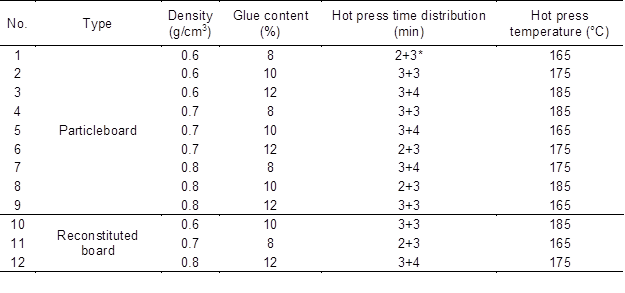*a+b: hot press temperature of secondary step-down pressure method; a: high pressure time; b: low pressure time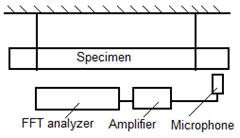Fig. 1. Flexural vibration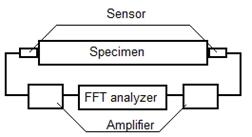Fig. 2. Longitudinal transmission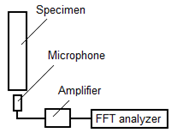Fig. 3. Longitudinal vibration

Longitudinal transmission test

Two sensors were placed on either end of the specimen: one to detect the transmitting signal, the other to detect the received signal. The transient impact on one end of the specimen was an emission signal. The receiver on the other end of the specimen received the vibrations from the emission signal and transmitted them through the amplifier to the FFT analyzer. The time difference between the emission and the reception of the signal was measured, and the relationship between sound velocity (c) and dynamic MOE (Ev) was used to calculate Ev,

Ev ρc(1)

where ρ is the density of specimen. The test set-up is shown in Fig. 2.

Longitudinal vibration test

In the longitudinal vibration test, the middle of the specimen was held between two fingers, and one end of the specimen was tapped. The generated signal was received by a microphone near the other end of the specimen, and the amplified signal was transmitted through the FFT analyzer. To calculate dynamic MOE (Ep), the longitudinal vibration frequency (fn) was used in the following formula,

Ep ρ(2Lfn/n)2 (2)

where ρ is the density of the specimen, L is the specimen length, and = 1, 2, 3,… The test set-up is shown in Fig. 3.

Destructive bending test

Based on the Chinese national standard GB/T17657-1999, a three-point bending destructive test using a SANS-CMT5504 universal mechanical testing machine was adapted in order to obtain the static MOE (Eb) and MOR (σb), after NDT (nondestructive technology) experiments.

RESULTS AND DISCUSSION

Table 2. Experimental Data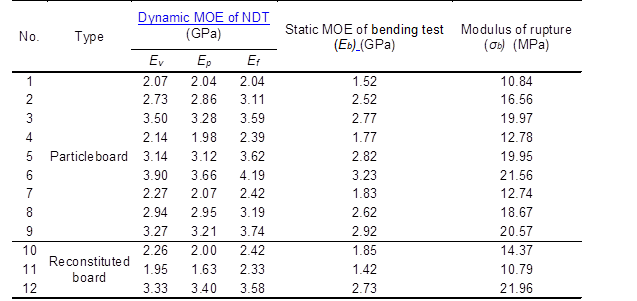Test Results

The data from NDT and the destructive bending test for the 12 types of specimens are shown in Table 2. The relationship between the dynamic MOE results of flexural vibration, longitudinal transmission, and longitudinal vibration tests and the three-point bending destructive test (static MOE) are shown in Fig. 4. From Fig. 4 and Table 2, it can be seen that the MOE values obtained from the nondestructive testing methods were greater than those obtained from the destructive bending method. Also among all of the NDT methods, the Ef derived from the flexural vibration method was the largest.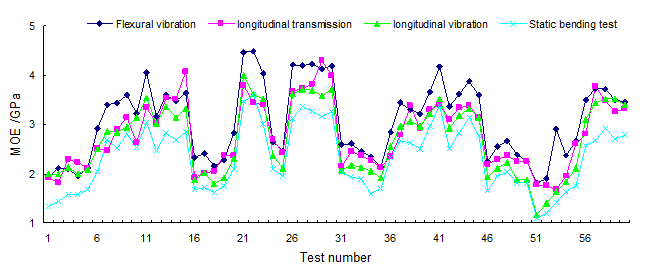Fig. 4. Static and dynamic MOE

Regression Analysis

Table 3. Linear Regression Formula and Regression Coefficient between Various Dynamic and Static MOEs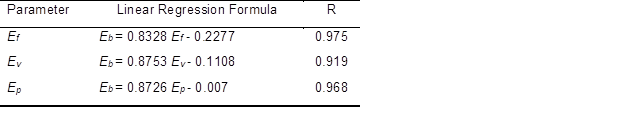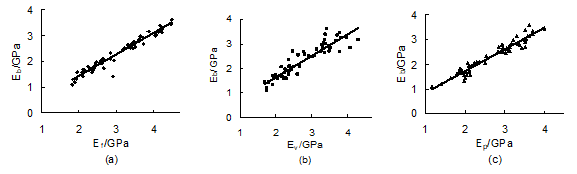Fig. 5. Correlation by regression curve between the dynamic and static MOEs

To obtain the relationship between various dynamic and static MOEs, regression analysis was conducted on the mechanical test results. The regression correlations between various dynamic and static MOEs are shown in Fig. 5, and the linear regression formula and regression coefficients are presented in Table 3. The results indicated that all the regression coefficients were much greater than R58,0.001 = 0.414, so there was a strong linear correlation between various MOE and MOR. The regression relationships from the above analysis were statistically significant; therefore, EfEvEp, and Eb were clearly related.

Table 4. Linear Regression Formula and Regression Coefficient between Various MOEs and Static MORs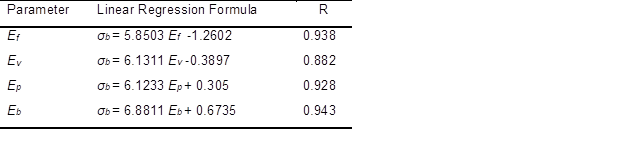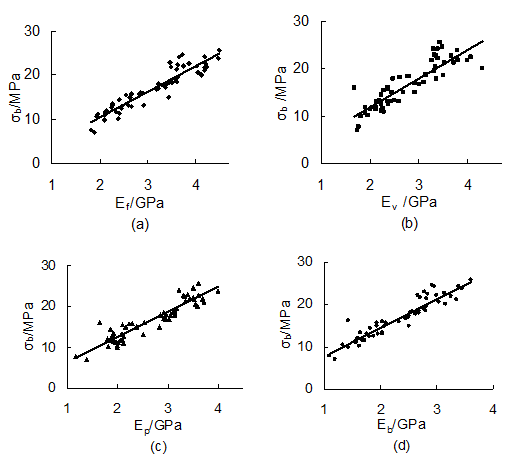Fig. 6. Correlation between dynamic MOE, static MOE, and static MOR

The relationship between various MOEs and static MORs can also be determined for the mechanical test results using regression analysis. The regression correlation between various MOEs (including static MOEs) and static MORs are shown in Fig. 6; the results demonstrated that, when using the flexural vibration test, longitudinal transmission test, or longitudinal vibration test to measure dynamic MOE, the results were highly correlated with MOR. The linear regression formula and regression coefficients are presented in Table 4. The results indicated that all the regression coefficients were much greater than R58,0.001 = 0.414, so there was also a strong linear correlation between various MOEs and MORs.

Through the above analyses, it was determined that the correlations between EfEp, and Ev (measured by FFT analysis) and Eb and σb were highly significant. The best correlation was between Ef and Eb, with an Rf = 0.975. The correlation with σ(of Ef and σb) was also good, with an Rf = 0.938. Therefore, the FFT NDT method can be fully applied in a feasible and accurate manner in the prediction of the MOE and MOR of soybean stalk particleboard and reconstituted board.

Performance Prediction Model

Taking flexural vibration as an example, the MOR can be predicted and the quality grade of boards can be classified using the following method. By using the linear correlation between Efand σb and considering the confidence of prediction, the regression equation can be changed to,

σaE± Δ (3)

where a and b are regression coefficients that are indexed in Table 4. Δ is the prediction error when the confidence of prediction is 95% (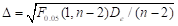), De is the deviation square sum of the measurement values,is the F test, and Δ is obtained from the Ef data.

Using Eq. (3), the lower and upper limits of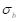are σb1 aEf1 + Δ and σb2 aEf2 – Δ, respectively. When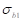and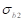can be determined, Ef1 and Ef2 can also be solved.

Calculating the measured test data of flexural vibration method and looking up the related data, the Δ result is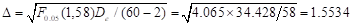. Using the regression coefficients in Table 4 and the Δ value in Eq. (3), σ= 5.8503 Ef – 1.2602 ± 1.5534.

According to the China national standard GB/T 20241-2006 for laminated veneer lumber (LVL), the MOR interval of the top-quality 60E LVL is [19.0, 22.5] MPa. Therefore, the range interval [σb1, σb2] = [19.0, 22.5] can be used to assess the quality grade of soybean stalk board. By introducing σb1 and σb2 into Eq. (3), Ef1 =3.20 GPa and Ef2 =4.33 GPa can be obtained, the interval [3.20, 4.33] was regarded as the MOR quality level. Samples 3, 5, 6, 9, 12, as seen in Table 2, can meet this interval; also, their MOR values were in the range of [19.0, 22.5] MPa, which is proof that the judgment method is reasonable.

Samples 3, 5, 6, 9, and 12 of the soybean stalk board can be judged as high-quality samples which can reach the quality demands of 60E LVL reference specimens, and the corresponding preparation technology can be used to guide the industrial production of soybean stalk board. Thus, dynamic MOE can be used to make a quality assessment based on truly nondestructive testing. This assessment method can also be adopted by the other two NDT methods: the longitudinal transmission and longitudinal vibration methods.

CONCLUSIONS

1. The values of dynamic MOE obtained by the fast Fourier transform (FFT) NDT were all greater than the values obtained by destructive bending tests in the experiment. The correlation between the dynamic and static MOEs was highly significant, the correlation between the dynamic MOEs measured by the flexural vibration test and the static MOE was the most significant. The correlation between the dynamic MOEs and MOR was also highly significant. Also, the correlation between the dynamic MOEs measured by the flexural vibration test and MOR was the most significant.
2. Through regression analysis of the MOR and dynamic MOEs, a prediction model and the level-determining interval could be obtained. This method can be effectively used to predict the performance and to determine the grade of soybean stalk board.
3. Because the processing capability of straw composites is poor, it is difficult to ensure satisfactory product quality. FFT analysis can be applied to quality testing of crop straw composites, but the application in actual production must be perfected in the future to guarantee sufficient quality.

ACKNOWLEDGMENTS

This project was funded by the National Natural Science Foundation of China (51203061), the Heilongjiang Educational Office Scientific Research Program (12531677) and Heilongjiang province scientific Brainstorm Program (GC12C204).

REFERENCES CITED

Hu, Y. C. (2004). The Dynamic Properties and Nondestructive Testing of Wood-based Composites Materials, Northeast For. Univ. Press, Harbin, China.

Hu, Y. C., Nakao, T., Nakai, T., Gu, J. Y., and Wang, F. H. (2005). “Dynamic properties of three types of wood-based composites,” J. Wood Sci. 51(1), 7-12.

Li, K. F., and Zhang, Z. G. (1992). “Process research of soybean stalk board,” Wood Process. Mach. 1, 20-24.

Reddy, N., and Yang, Y. (2009). “Natural cellulose fibers from soybean straw,” Bioresour. Technol. 100(14), 3593-3598.

Shi, Y. L. (2006). “Studies on experiment of process characteristic of mix-material flakeboard,” MS Thesis, Jilin Agricultural University, China.

Song, X. Z., Chen, D., Guo, K. Q., and Zhang, B. J. (2008). “Technical analysis and prospect of crop straw scrimber,” J. Northwest A & F Univ. 36(5), 229-234.

Xing, C., Deng, Y. H., and Yin, S. Z. (1999). “Manufacture technology of particleboard from soybean stalk,” China Forestry Science and Technology (2), 21-23.,

Yu, M., Ren, H. E., and Bai, F. (2005). “Method research of measuring timber intensity without damage,” For. Mach. Woodwork. Equip. 33(2), 35-39.

Zhou, Y. (1997). “Preliminary study on manufacture technology of particleboard from soybean stalk,” Wood Ind. 11(4), 15-17.

Article submitted: May 16, 2013; Peer review completed: June 26, 2013; Revised version accepted: July 25, 2013; Published: July 31, 2013.